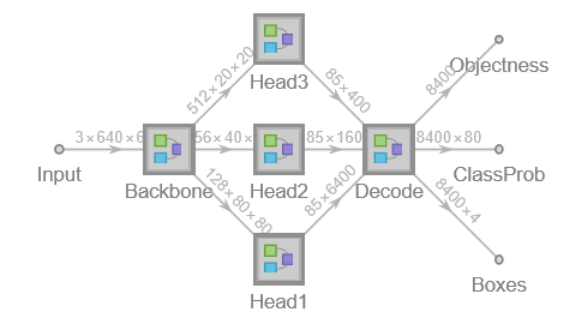# YOLOX Trained onMS-COCO Data

Detect and localize objects in an image

YOLO (You Only Look Once) Version X is a family of object detection models published in August 2021. It is a revisitation of the YOLOv3-DarkNet53 model with several architectural and training improvements: the decoupling of classification and regression heads, the switch to an anchor-free pipeline, the introduction of an advanced label-assignment strategy named SimOTA (Simplified Optimal Transport Assignment) and the use of strong data augmentation techniques.

## Training Set Information

• Microsoft COCO, a dataset for image recognition, segmentation and captioning, consisting of more than three hundred thousand images overall consisting of 80 object classes.

## Model Information

•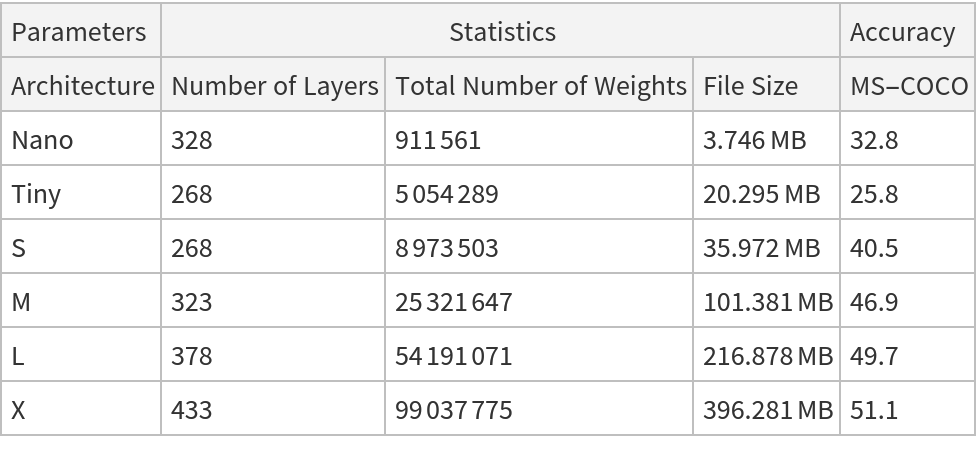## Examples

### Resource retrieval

Get the pre-trained net:

 In:=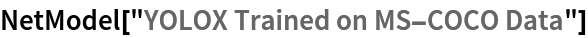Out=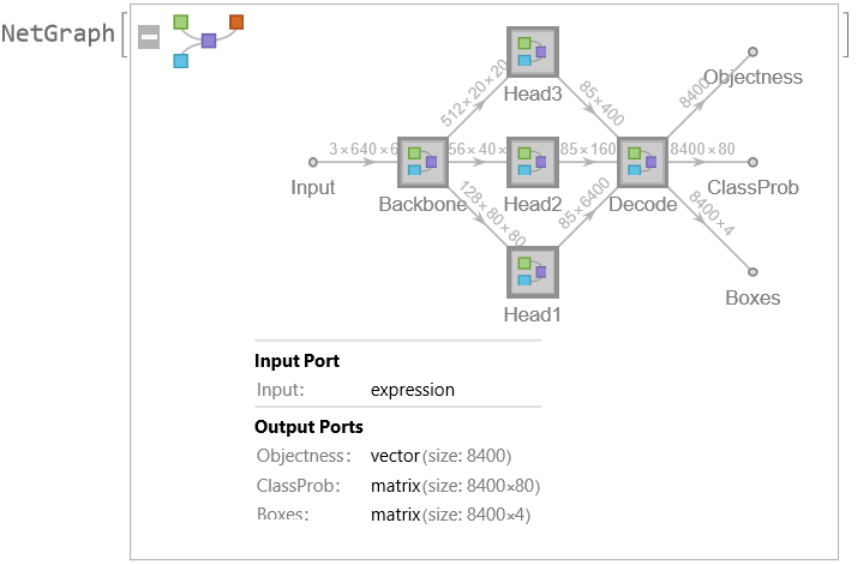### NetModel parameters

This model consists of a family of individual nets, each identified by a specific parameter combination. Inspect the available parameters:

 In:=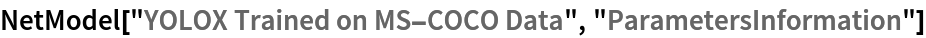Out=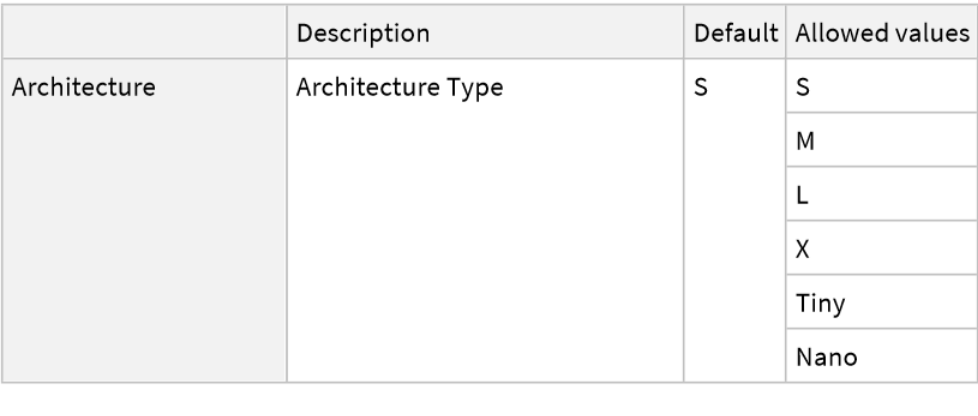Pick a non-default net by specifying the parameters:

 In:=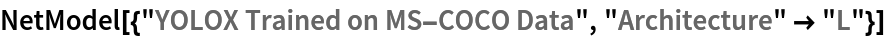Out=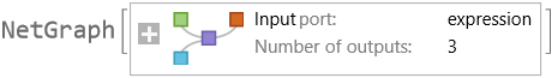Pick a non-default uninitialized net:

 In:=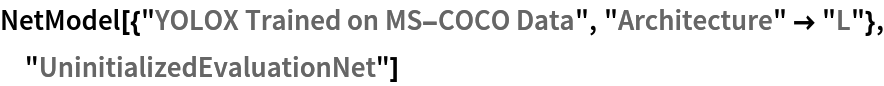Out=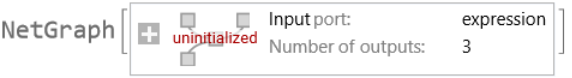### Evaluation function

Write an evaluation function to scale the result to the input image size and suppress the least probable detections:

 In:=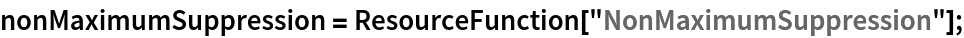In:=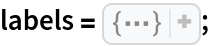In:=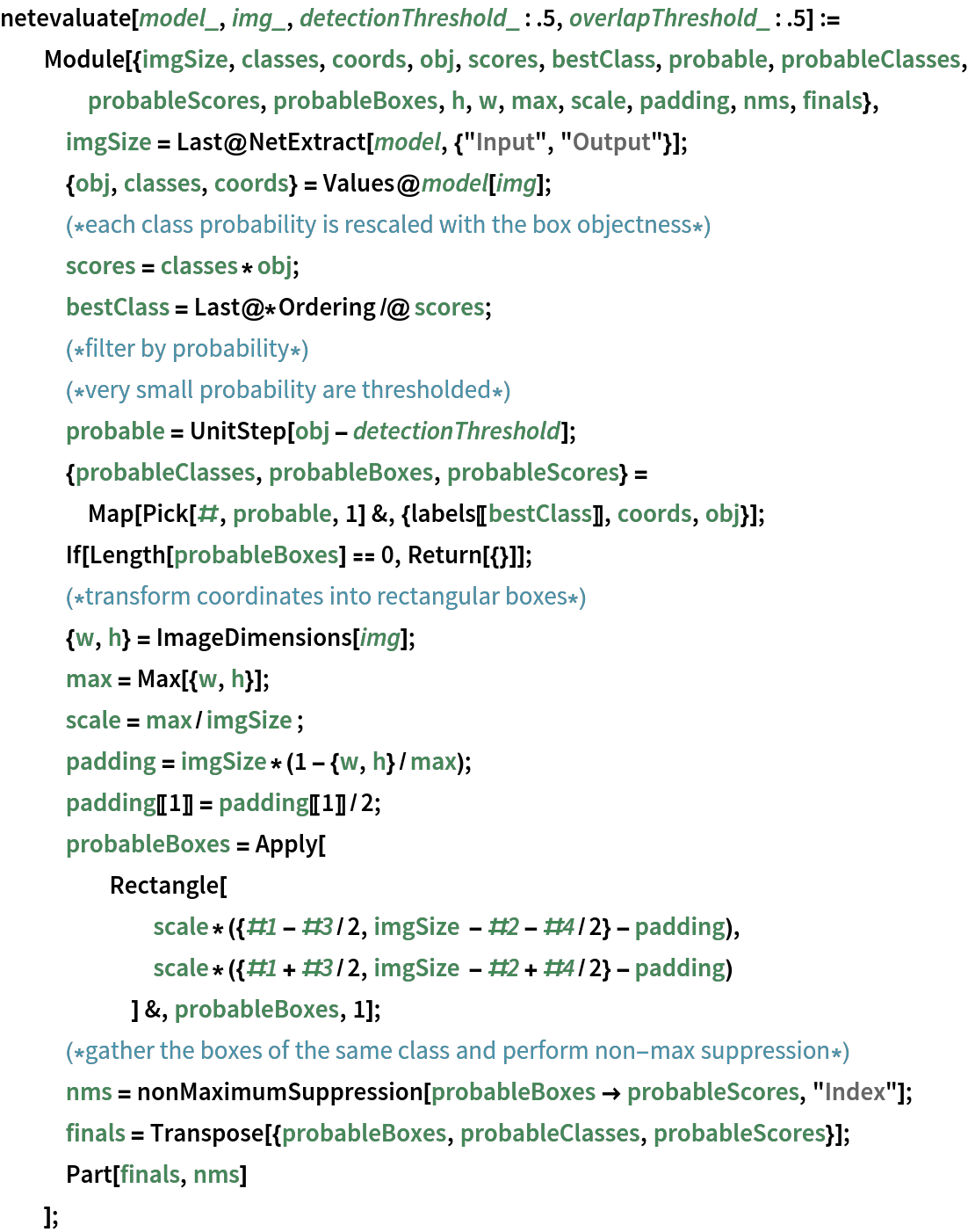### Basic usage

Obtain the detected bounding boxes with their corresponding classes and confidences for a given image:

 In:=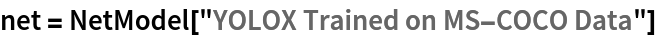Out=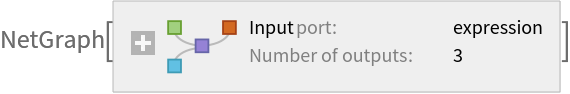In:=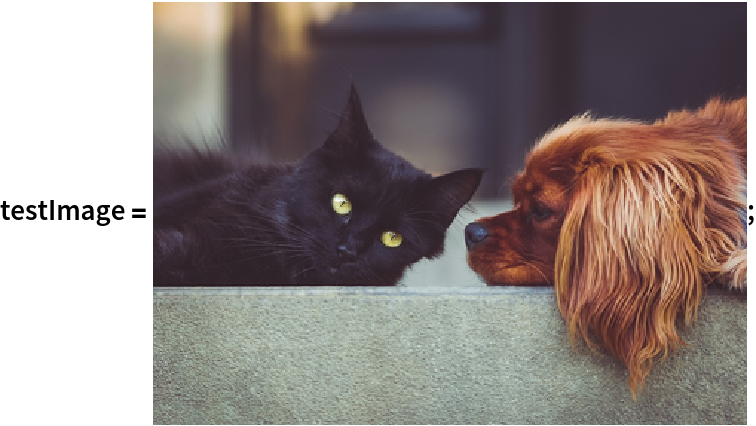In:=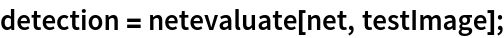Inspect which classes are detected:

 In:=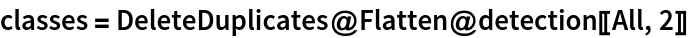Out=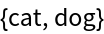Visualize the detection:

 In:=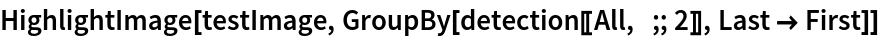Out=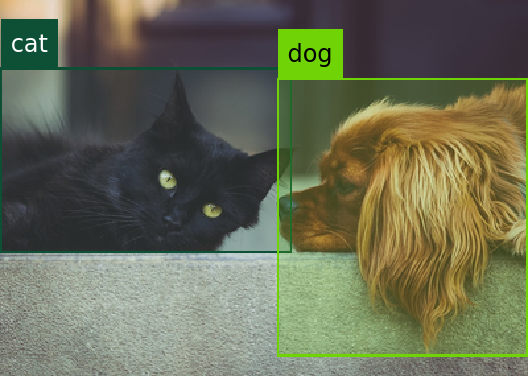### Network result

The network computes 8,400 bounding boxes and the probability that the objects in each box are of any given class:

 In:=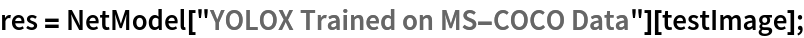In:=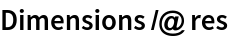Out=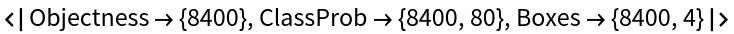Rescale the bounding boxes to the coordinates of the input image and visualize them scaled by their "objectness" measures:

 In:=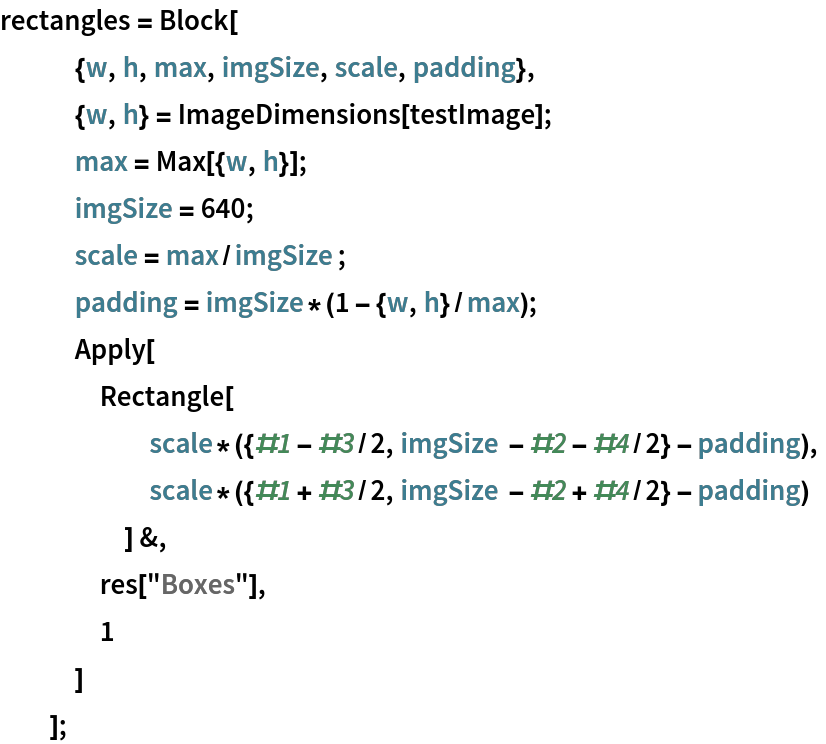In:=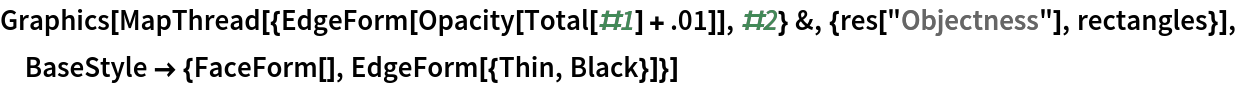Out=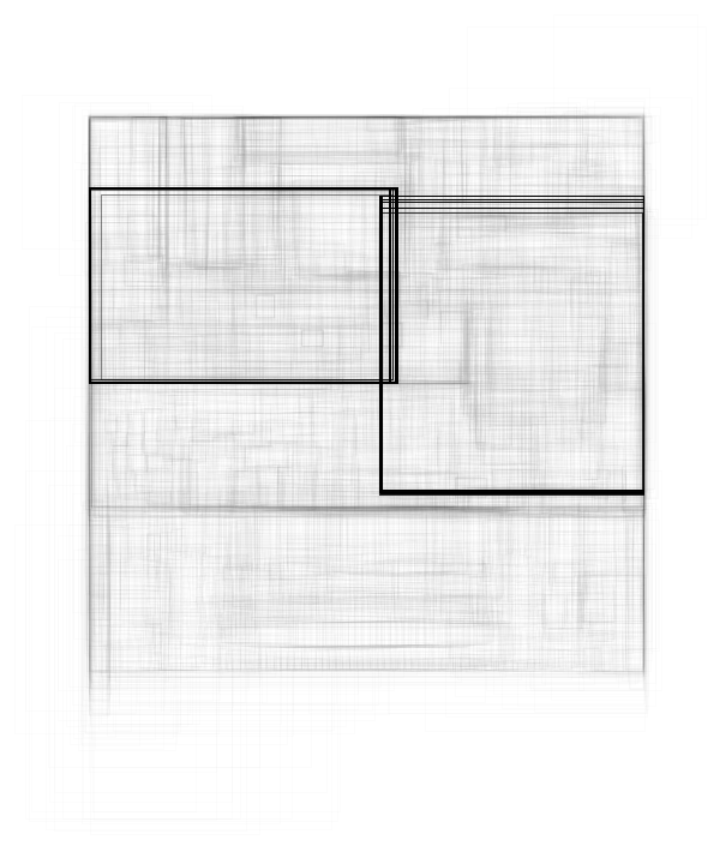Visualize all the boxes scaled by the probability that they contain a cat:

 In:=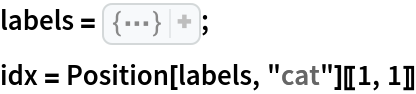Out=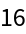In:=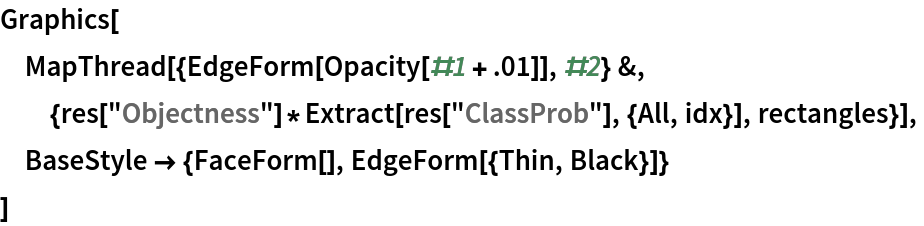Out=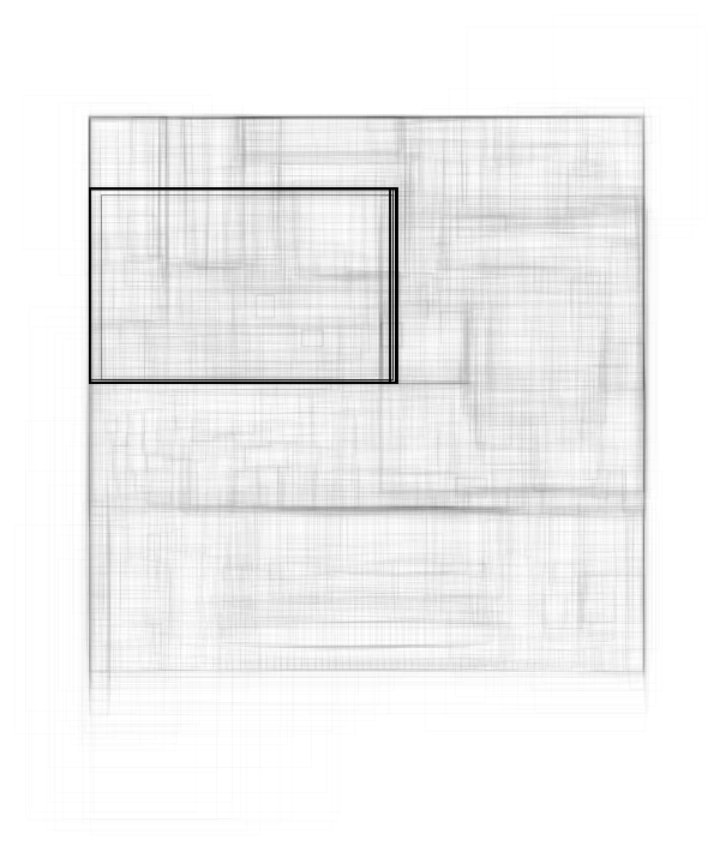Superimpose the cat prediction on top of the scaled input received by the net:

 In:=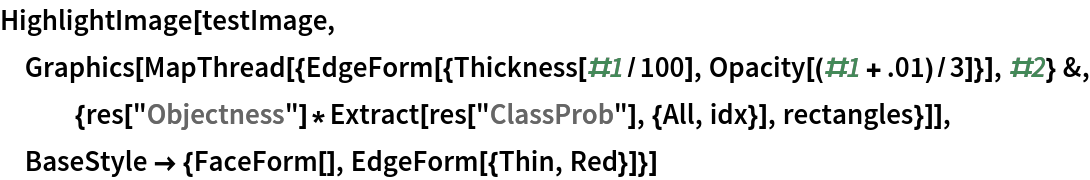Out=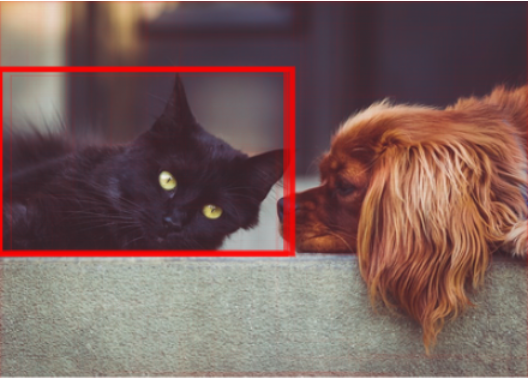### Net information

Inspect the number of parameters of all arrays in the net:

 In:=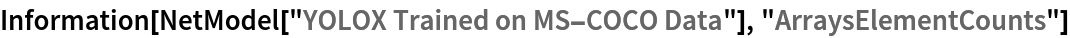Out=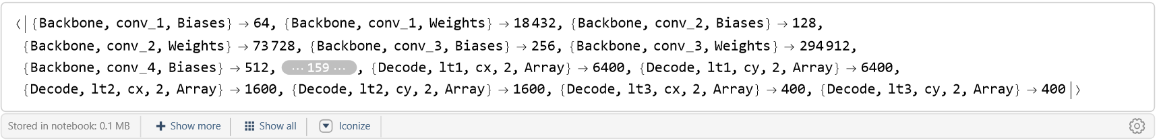Obtain the total number of parameters:

 In:=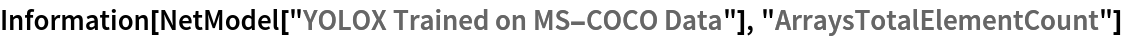Out=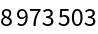Obtain the layer type counts:

 In:=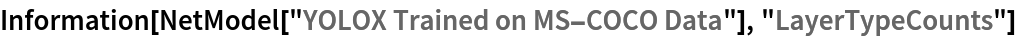Out=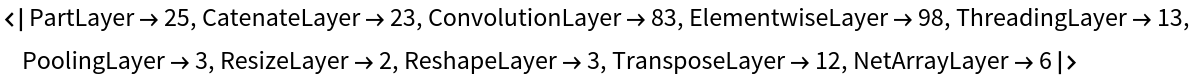Display the summary graphic:

 In:=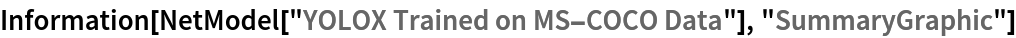Out=